# Step Change in a proportional plus integral controller

Gold Member

## Homework Statement

Figure 5 shows the input and output wave forms for a proportional plus integral controller. State:
(i) the controllers proportional gain
(ii) the controllers integral action time

The attachment below is a copy and paste of the input and output wave forms I am given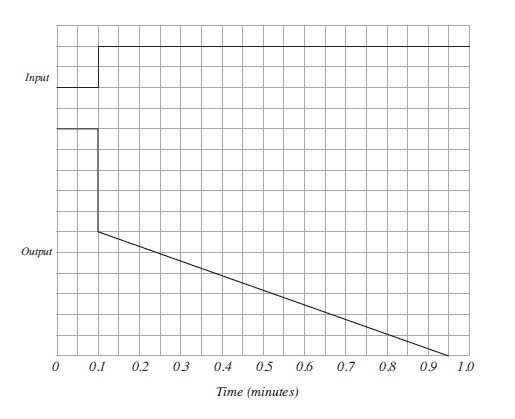## Homework Equations

[/B]
I use the equation ##gain = \frac{100}{\%PB}##

Integral action time is the time taken for the initial change due to proportional action to be repeated by the integral action when the input to the controller is subject to a step change

## The Attempt at a Solution

I[/B] am looking at where to start with this as all of my lessons show the output moving in a positive direction (up) so do I assume this is some kind of reverse acting device as the output is moving down as the input increases.
There are no values given on the y-axis so I do not know ranges etc. In a situation like this, if I input my own values would should I still be able to calculate the correct values? I am just looking for a little bit of info to initially start me off in the correct direction

#### Attachments

Gold Member
Dearly Missed
I am just looking for a little bit of info to initially start me off in the correct direction

Well you have to work with what you're given.

I see input stepping UP two squares
and output stepping DOWN five squares
so i'd answer gain is -5/2 squares per square which gives gain = -2.5 and the units cancel.
Controls has its own jargon and that's not even consistent between manufacturers
often the negative sign is taken care of by the word "reverse"
and PB is pretty universally inverse of gain expressed in %

What (if any) units for gain did instructor use in class ?

•FactChecker
Homework Helper
Gold Member
Don't know what "PB" stands for but I agree with post 2 re: the proportional gain.
So how about the integral gain?

The units don't matter; you can just think of the units as dimensionless, or volts, or whatever.

Gold Member
PB stands for proportional band. We were not given any units for gain so as you say its just a number. This has given me something to work on, thanks

Gold Member
Dearly Missed
So how about the integral gain?

Some manufacturers apply proportional gain to integral action, some do not.
It depends on whether or not their internal error term gets multiplied by gain before applying integral.

In his graph,
output wasn't integrating before the step change at input, so error then must have been zero.
After the step we can assume there's an error of two squares.
Output changes by an amount equal to error, 2 squares, in 0.28 minutes so that'd be the integral time in some makes of controller.
Output changes by an amount equal to error X gain, 5 squares, in 0.7 minutes so that'd be the integral time in other makes of controller.
Which did his textbook assume? He didn't say.
In my experience the latter is more common but far from ubiquitous.
You'll also often see "Integral action" named "Reset" instead ...

old jim

Gold Member
I dug deeper into the lessons and discovered another method of calculating the gain which makes far more sense and agrees totally with Jims post number 2

##gain=\frac{output change}{input change}## so ##gain =\frac{-5}{2}=-2.5##

I also found an example of the Integral action time in one of the lessons and its explained quite well (I need to read more into these lessons) I have attached an extract from the lesson below.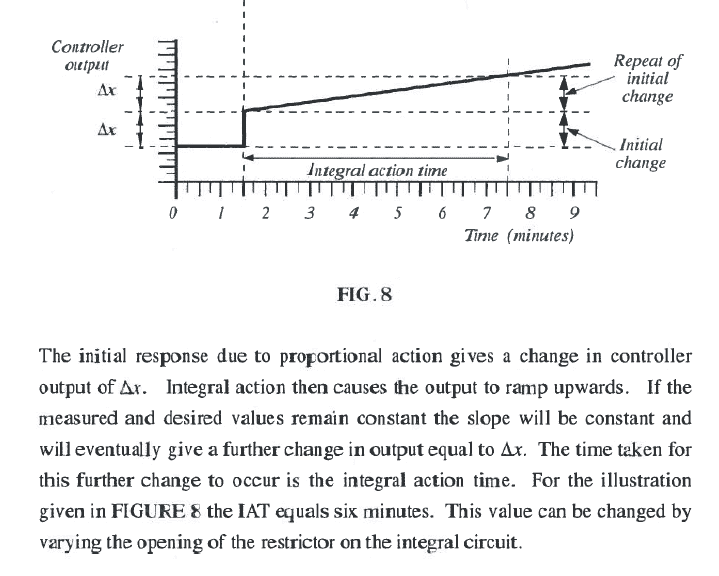Based on the above I am certain that the correct value for Question (ii) will be 0.7 seconds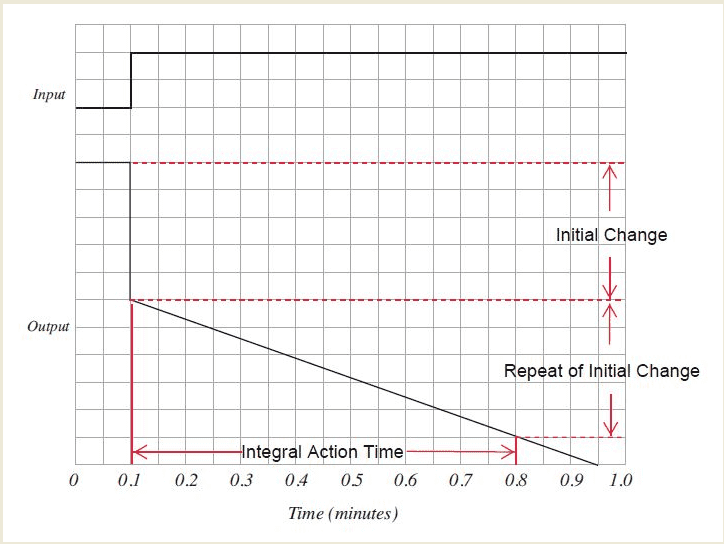Going back to my first post I was wondering about such things as values on y-axis etc but they were never required for this question.

I think I am ok for part (a)(i and ii) of the question for now so thank you once again and I will move onto part B which is :-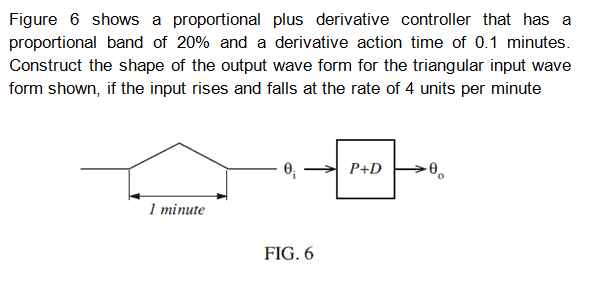#### Attachments

Gold Member
Dearly Missed
"...opening the restrict or on the integral circuit..." ??
Wow that sounds like a pneumatic controller - you'll find excellent training materials in old Bailey and Fisher literature .

Integral of a constant is a ramp , ∫kdt = kt (plus Constant of integration) and C is just whatever output was when integration began

Derivative is also called "Rate"
and as name implies it's derivative of error

your ramp is a constant rate of change so derivative will be its rate of change .

Gold Member
Dearly Missed
i love it !These mechanical analog computers were well suited to boiler control.
Oblivious to power line spikes from lightning, and impervious to EMP attack.
From a slight touch on the beam you could judge their health by the 'hiss' .

#### Attachments

Gold Member
Going back to post 6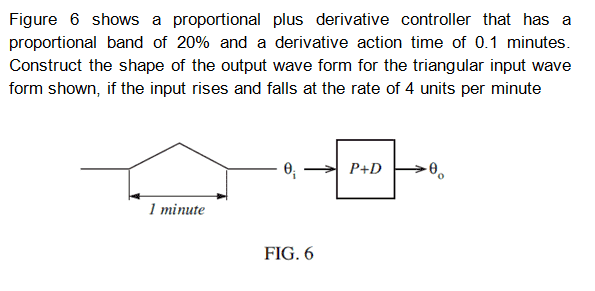This question only asks me to actually create a waveform. If it rises and falls over 4 units per minute then it must start at zero and reach its peak after 2 units then back down to zero when it reaches 4 units. As shown in the drawing above.

I have made a drawing below of what I think the output wave form should look like based on limited info I have in the lesson notes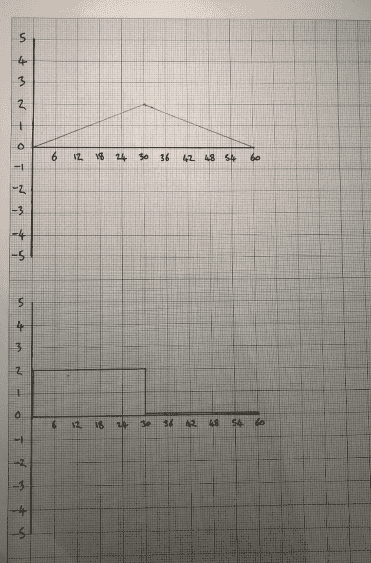Does this make any sense??

#### Attachments

Gold Member
Hello again, just a thanks for the help with this, I submitted my answers and everything in this thread was confirmed as correct. Many thanks

Gold Member
Dearly Missed

I have made a drawing below of what I think the output wave form should look like based on limited info I have in the lesson notesyour plot looks almost right for the derivative part
You could consider the two terms as separate computations then sum them.

derivative term should be the slope of input so steps at first positive then negative

and that is added to the proportional term , which i don't see represented in your second line

old jim

#### Attachments

Jason-Li
Going back to post 6

View attachment 234947

This question only asks me to actually create a waveform. If it rises and falls over 4 units per minute then it must start at zero and reach its peak after 2 units then back down to zero when it reaches 4 units. As shown in the drawing above.

I have made a drawing below of what I think the output wave form should look like based on limited info I have in the lesson notes

View attachment 234946

Does this make any sense??

Hi DavidJ,

Sorry to resurrect an old post. Was this marked correct? From what I can tell it doesn't have the derivative action drawn?

Gold Member
Hello Jason

This was marked as correct. Its a long time ago now. I had actually submitted this for marking before Jim pointed out about the proportional but thinking back I did ask about this and was told it was not required

Jason-Li
Hello Jason

This was marked as correct. Its a long time ago now. I had actually submitted this for marking before Jim pointed out about the proportional but thinking back I did ask about this and was told it was not required

Hi David thanks for the reply. The questions just seem so vague sometimes! Thanks again.